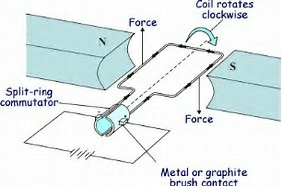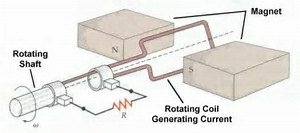# Electric Motor

• Electrical energy is converted into mechanical energy by using an electric motor.
• Electric motor works on the basis of rule suggested by Marie Ampere and Fleming’s Left Hand Rule.
• In an electric motor, a rectangular coil is suspended between the two poles of a magnetic field. The electric supply to the coil is connected with a commutator. Commutator is a device which reverses the direction of flow of electric current through a circuit.
• When electric current is supplied to the coil of electric motor, it gets deflected because of magnetic field.
• As it reaches the half way, the split ring which acts as commutator reverses the direction of flow of electric current. Reversal of direction of current reverses the direction of forces acting on the coil.
• The change in direction of force pushes the coil, and it moves another half turn. Thus, the coil completes one rotation around the axle. Continuation of this process keeps the motor in rotation.
• In commercial motor, electromagnet instead of permanent magnet and armature is used.
• Armature is a soft iron core with large number of conducting wire turns over it. Large number of turns of conducting wire enhances the magnetic field produced by armature.# Michael Faraday law of Electromagnetic Induction

When a conductor is set to move inside a magnetic field or a magnetic field is set to be changing around a conductor, electric current is induced in the conductor.

# Galvanometer

It is an instrument that can detect the presence of a current in a circuit.

If pointer is at zero (the centre of scale) then there will be no flow of current. If the pointer deflects on either side right or left, this will show the direction of current. Represented by# Electric Generator

The structure of electric generator is similar to that of an electric motor. In case of an electric generator a rectangular armature is placed within the magnetic field of a permanent magnet.

• The armature is attached to wire and is positioned in way that it can move around an axle. When the armature moves within the magnetic field an electric current is induced.
• The direction of induced current changes, when the armature crosses the halfway mark of its rotation. Thus, the direction of current changes once in every rotation. Due to this, the electric generator usually produces alternate current, i.e. AC.
• To convert an AC generator into a DC generator, a split ring commutator is used. This helps in producing direct current.# Advantages of Alternate Current (AC) over Direct Current (DC)

Electric power can be transmitted to longer distances without much loss of energy. Therefore, cost of transmission is low.

In India the frequency of AC is 50Hz. It means after every 1/100 second it changes its direction.